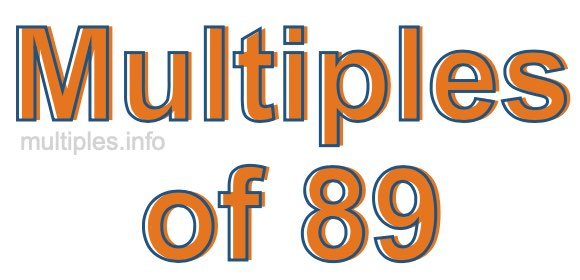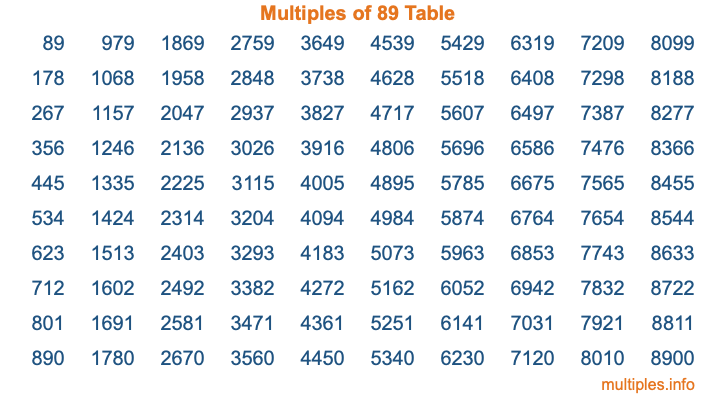Multiples of 89Welcome to the Multiples of 89 page. Here we will first teach you everything you will ever need to know about the multiples of 89, and then give you a study guide summary of everything we taught you to make sure you remember it all. Use this page to look up facts and learn information about the multiples of 89. This page will make you a multiples of eighty-nine expert!

Definition of Multiples of 89
Multiples of 89 are all the numbers that when divided by 89 equal an integer. Each of the multiples of 89 are called a multiple. A multiple of 89 is created by multiplying 89 by an integer.

Therefore, to create a list of multiples of 89, you start with 1 multiplied by 89, then 2 multiplied by 89, then 3 multiplied by 89, and so on for as long as you want. Thus, the list of the first five multiples of 89 is 89, 178, 267, 356, and 445. To see a larger list of multiples of 89, see the printable image of Multiples of 89 further down on this page. We also have a category where you can choose any nth multiple of 89.

Multiples of 89 Checker
The Multiples of 89 Checker below checks to see if any number of your choice is a multiple of 89. In other words, it checks to see if there is any number (integer) that when multiplied by 89 will equal your number. To do that, we divide your number by 89. If the the quotient is an integer, then your number is a multiple of 89.

Is  a multiple of 89?

Least Common Multiple of 89 and ...
A Least Common Multiple (LCM) is the lowest multiple that two or more numbers have in common. This is also called the smallest common multiple or lowest common multiple and is useful to know when you are adding our subtracting fractions. Enter one or more numbers below (89 is already entered) to find the LCM.

Check out our LCM Calculator if you need more details about the Least Common Multiple or if you need the LCM for different numbers for adding and subtraction fractions.

nth Multiple of 89
As we stated above, 89 is the first multiple of 89, 178 is the second multiple of 89, 267 is the third multiple of 89, and so on. Enter a number below to find the nth multiple of 89.

th multiple of 89

Multiples of 89 vs Factors of 89
89 is a multiple of 89 and a factor of 89, but that is where the similarities end. All postive multiples of 89 are 89 or greater than 89. All positive factors of 89 are 89 or less than 89.

Below is the beginning list of multiples of 89 and the factors of 89 so you can compare:

Multiples of 89: 89, 178, 267, 356, 445, etc.

Factors of 89: 1, 89

As you can see, the multiples of 89 are all the numbers that you can divide by 89 to get a whole number. The factors of 89, on the other hand, are all the whole numbers that you can multiply by another whole number to get 89.

It's also interesting to note that if a number (x) is a factor of 89, then 89 will also be a multiple of that number (x).

Multiples of 89 vs Divisors of 89
The divisors of 89 are all the integers that 89 can be divided by evenly. Below is a list of the divisors of 89.

Divisors of 89: 1, 89

The interesting thing to note here is that if you take any multiple of 89 and divide it by a divisor of 89, you will see that the quotient is an integer.

Multiples of 89 Table
Below is an image of the first 100 multiples of 89 in a table. The table is in chronological order, column by column. The first column has the first ten multiples of 89, the second column has the next ten multiples of 89, and so on.The Multiples of 89 Table is also referred to as the 89 Times Table or Times Table of 89. You are welcome to print out our table for your studies.

Negative Multiples of 89
Although not often discussed or needed in math, it is worth mentioning that you can make a list of negative multiples of 89 by multiplying 89 by -1, then by -2, then by -3, and so on, to get the following list of negative multiples of 89:

-89, -178, -267, -356, -445, etc.

Multiples of 89 Summary
Below is a summary of important Multiples of 89 facts that we have discussed on this page. To retain the knowledge on this page, we recommend that you read through the summary and explain to yourself or a study partner why they hold true.

There are an infinite number of multiples of 89.

A multiple of 89 divided by 89 will equal a whole number.

89 divided by a factor of 89 equals a divisor of 89.

The nth multiple of 89 is n times 89.

The largest factor of 89 is equal to the first positive multiple of 89.

89 is a multiple of every factor of 89.

89 is a multiple of 89.

A multiple of 89 divided by a divisor of 89 equals an integer.

89 divided by a divisor of 89 equals a factor of 89.

Any integer times 89 will equal a multiple of 89.

Multiples of a Number
Here you can get the multiples of another number, all with the same attention to detail as we did for multiples of 89 on this page.

Multiples of
Multiples of 90
Did you find our page about multiples of eighty-nine educational? Do you want more knowledge? Check out the multiples of the next number on our list!